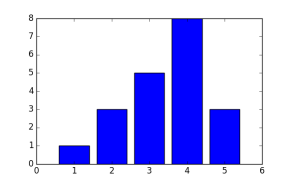# Prime factors, phone numbers, and the normal distribution

Telephone numbers typically have around three distinct prime factors.

The length of a phone number varies by country, but US a phone number is a 10 digit number, and 10-digit numbers are often divisible by three different prime numbers, give or take a couple. Assuming phone numbers are scattered among possible 10-digit numbers in a way that doesn’t bias their number of prime factors, these numbers will often have between 1 and 5 prime factors. If a country has 9- or 11-digit phone numbers, the result is essentially the same.

Let ω(n) be the number of distinct prime factors of n. Then the Erdős–Kac theorem says roughly that ω(n) is distributed like a normal random variable with mean and variance log log n. More precisely, fix two numbers a and b.  For a given value of x, count the proportion of positive integers less than x where

(ω(n) – log log n) / sqrt( log log n)

is between a and b. Then in the limit as x goes to infinity, this proportion approaches the probability that a standard normal random variable is between a and b.

So by that heuristic, the number of distinct prime factors of a 10-digit number is approximately normally distributed with mean and variance log log 10^11 = 3.232, and such a distribution is between 1 and 5 around 73% of the time.

My business phone number, for example, is 8324228646. Obviously this is divisible by 2. In fact it equals 2 × 32 × 462457147, and so it has exactly three distinct prime factors: 2, 3, and 462457147.

Here’s how you could play with this using Python.

```    from sympy.ntheory import factorint

def omega(n):
return len(factorint(n))
```

I looked in SymPy and didn’t see an implementation of ω(n) directly, but it does have a function factorint that returns the prime factors of a number, along with their multiplicities, in a dictionary. So ω(n) is just the size of that dictionary.

I took the first 20 phone numbers in my contacts and ran them through `omega` and got results consistent with what you’d expect from the theory above. One was prime, and none had more than five factors.## 2 thoughts on “Prime factors, phone numbers, and the normal distribution”

1. Mike G

Fun… Seems to work out for my contacts as well.

My old cell phone # has 6 factors, while my new one is prime. But it’s still easier to remember the old #, dangit.

2. Torsten Seemann

Over the last years I scrutinized the distribution of prime factors. Hereinafter you find the distribution of the numbers of (distinct) prime factors for all numbers up to 10 billions:
1 455062599
2 1894770259
3 3183957558
4 2766436075
5 1319295741
6 336846913
7 41607092
8 2001071
9 22685
10 6

Indeed more than 96% have one to five prime factors.